# [Studyplan] SSC-CGL Maths, Quantitative Aptitude, Algebra, Trigonometry: Approach, Booklist, Strategy, Free Studymaterial 2013 for Combined Graduate Level Exam Tier 1, 2

In SSC by Support Staff

# introduction

Competitive exams are meant for real-men and women. This is no country for crybabies, kids, college teens and no0bs. So first of all, you must get rid of the following “loser” mindsets:

1. Yaar this maths is so hard, I can’t do it.
2. I’m not from science/engineering background hence this is not my cup of tea.
3. I’m poor in maths and I cannot improve.
4. Thik hai, dekh lenge. (alright, I’ll see).
• Maths is not difficult. All it requires is concept clarity + lot of practice. In SSC-CGL exam, you’ve to face Mathematics at two stages
 Stage Maths-Questions Penalty Tier-I (Prelims) 50 Qs Negative 0.25 Tier-II (Mains) Paper I: Arithmetical Ability 100 Qs worth 200 marks Negative 0.50
• The Approach for Maths, stands on two pillars.

## How?

1. Conceptual clarity
2. Mrunal.org/aptitude
3. For some topics, directly Quantitative aptitude books.
1. Lot of practice
• From Quantitative aptitude books.
• There are lot of books in market, the question is, which one to refer? It is explained at the bottom of this article.

# #1: Getting the conceptual clarity

• We’ll divide Maths or Quantitative Aptitude, into topics and further into subtopics.
• Your task is to cover one topic at a time, first get conceptual-clarity and then solve maximum questions at home.
• Whenever you learn any shortcut technique, you note it down in your diary.
• Similarly, whenever you make any mistake while solving sums, you also note that down in your diary. Night before the exam, you review that diary of mistakes. (why do this? Because it is the “Art of Aptitude” (Click ME)
Topic Subtopics How to approach

## Number theory

1. Divisibility, remainders
2. LCM and HCF
3. Unknown numbers from given conditiofor
4. Fractions-comparisions.
• NCERT Class 7 Chap 2, 9 (fraction)
• NCERT Class 10 Chap 1 (divisibility)
• Finally your Quantitative aptitude book.

## Basic Maths

1. Simplification (BODMAS)
• NCERT Class 8 Chap 1
1. Surds, indices
• NCERT Class 8 Chap 12
• Then NCERT Class 9 Chap 1
1. Roots, squares, Cubes
• Basics from NCERT Class 8 Chap 6 and 7.

## Algebra

1. Linear equation
• “Mother’s age was x and daughters age..”
• “3 mangos and 5 bananas purchased for…”
• X+1/2x+3=3/8 then find X.
• ^This type of stuff. Just practice and you’ll get a hang of it.
• Basics given in NCERT Class 8 Chap 2 and 9.
• Then NCERT Class 9 Chap 4
• Lastly NCERT Class 10 Chap 3.
1. Quadratic equations, Polynomials
• Factorization and roots. Heavily asked in Tier-II.
• NCERT Class 8 Chap 14
• And then NCERT Class 9 Chap 2
• Lastly NCERT Class 10 Chap 4

## Avg and Ratios

1. Wine-Water mixture (Alligations)
Can be solved without formula. Go through https://mrunal.org/2012/03/aptitude-alligationsmixturesalloys.html
1. Simple Average
Lolz
1. Ratio-Proportion-variations
For ratio-proportion NCERT Class 8 Chap 13.
1. Partnership
https://mrunal.org/2012/05/aptitude-partnership-and-profit-sharing.html

## STD

1. Time speed distance
2. Trains, platforms
3. Boats-streams
4. Time and Work
5. Pipes and Cisterns
All of them can be solved with just one Universal STD formula. Explained in www.Mrunal.org/aptitude

## Geometry

1. Angles, sides, bisectors, circles etc
• NCERT Class 9 Chap 6, 7, 8 and 10. Quantitative aptitude book.
1. Mensuration (area and volume).
• Basics explained in NCERT Class 8 Chap 3, 11.
• Then NCERT Class 9 Chap 9, 12, 13.
• Lastly Lastly NCERT Class 10 Chap 13
1. Trigonometry
Understand basics from NCERT Class 10 Chap 8 and 9. Then exam-oriented concepts from your Quantitative Aptitude book.

## %

• Basic % (increase, decrease in consumption, population)
Also do NCERT Class 8 Chap 8.
• Data-interpretation cases.
Mere extention of % concept. Just practice.
For long division, use this approximation method: https://mrunal.org/2012/11/aptitude-long-division-two-digit-division-calculation-without-tears-and-without-boring-vedic-speed-maths.html
Profit, loss, discount, marked price. https://mrunal.org/2012/11/aptitude-concepts-of-marked-price-and-successive-discounts-profit-loss-without-stupid-formulas.html
Simple and compound interest rate https://mrunal.org/2012/04/aptitude-compound-interest-rate.html

## PCP

1. Permutation
2. Combination
3. Probability
• Not asked as such.
• But Sometimes a question or two comes in the “Reasoning” portion of Tier-I. Hence not much attention necessary.
• But again, they too can be solved without mugging up formulas: go through various articles on www.Mrunal.org/aptitude

## Misc.

1. Coordinate Geometry
• Lately SSC has started asking 1-2 question in each of Tier I and II. But they can be solved by merely plugging numbers in the readymade formulas given in your Quantitative Aptitude book.
1. Progression: Arithmetic+ Geometry
• Again, rarely asked in SSC. Sometimes a question or two comes in the “Reasoning” portion of Tier-I.
• Basically you just have to plug in the values in formulas. Basics of Arithmetic progression explained in NCERT Class 10 Chap 5.
1. Logerithms

# Breakup: SSC-CGL Tier I (2010, 2011, 2012)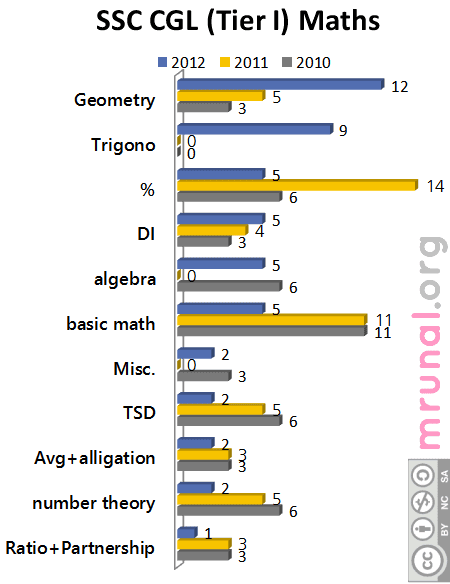Topic 2010 2011 2012
Ratio+Partnership 3 3 1
number theory 6 5 2
Avg+alligation 3 3 2
TSD 6 5 2
Misc. 3 0 2
basic math 11 11 5
algebra 6 0 5
DI 3 4 5
% 6 14 5
Trigonometry 0 0 9
Geometry 3 5 12
Total 50 50 50

The 2012’s Tier-I paper, is “trend-breaker”. Because

1. The conventional questions from ratio-proportion, basic maths, time-speed-work are asked for namesake only.
2. Otherwise, Out of 50 Maths questions in tier-I, almost 30 questions are from just Geometry+Trig+Percentage application.
3. Earlier, they used to ask mostly area-volume-perimeter type questions from Geometry segment. You just had to plug-in values into the formulas and get the answer.
4. But This 2012’s paper has mostly theory based geometry (angle, bisector, tangent, inequality of triangles etc.)
5. Similarly the difficulty level of algebra, number theory based questions is bit raised.

Or perhaps SSC too decided to employ the BackbreakingTM move of UPSC! Anyways, jokes apart, the lesson here is, “adapt.”

# Breakup: SSC-CGL Tier II (2010, 2011, 2012)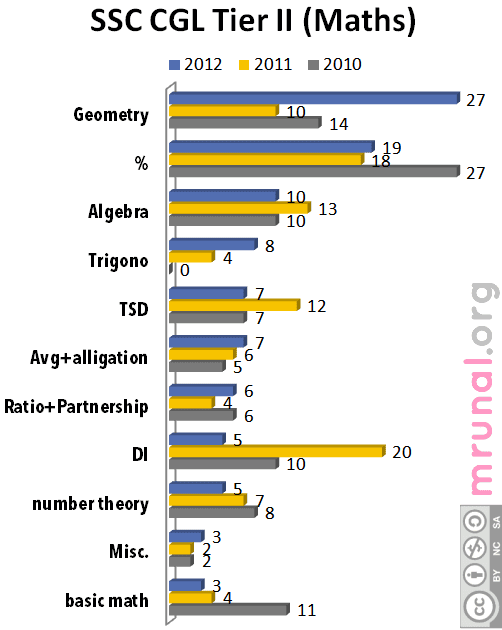Type 2010 2011 2012
basic math 11 4 3
Misc. 2 2 3
number theory 8 7 5
DI 10 20 5
Ratio+Partnership 6 4 6
Avg+alligation 5 6 7
TSD 7 12 7
Trigonometry 0 4 8
Algebra 10 13 10
% 27 18 19
Geometry 14 10 27
Total 100 100 100
• Here too, Geometry+Trigonometry have been given emphasis like never before.
• Almost 65% of the paper is made up of Geometry, Trig, Percentage and Algebra (and in that too, mostly Quadratic equations.)

# #2: Practice

• Merely knowing the concepts or formulas won’t help. Because unless you practice different variety of questions, you won’t become proficient in applying those concepts flawlessly in the actual-exam.
• Second, despite knowing concept and formulas, people make silly mistakes either in calculation or in pluging the values.
• Third reason- Tier I has 200 questions in 120 limits. =not even 2 minutes per question. Plus, questions reasoning and comprehension might take more than 5 minutes! Therefore speed is essential. Since there is negative marking system, accuracy also matters.
• So it is beyond doubt that you have to practice excimer number of questions at home.
• The question is where to get the practice? Which book should be used for SSC exam?

# Choice of Quantitative Aptitude Book?

• In all competitive exams, “uncertainity factor” is involved. Despite your best preparation, you might lose the success-train by 2-3 marks.
• Therefore you must never put all eggs in one basket.
• While you are preparing for SSC, you should also keep open mind and apply for other competitive exams, such as IBPS, ACIO, ONGC, Railways, LIC, CDS, Coast Guard etc. (Depending on your career-taste).
• Publication houses will come up with new books for each and every of ^these exams, but we have neither the time nor the money to buy a new book for every new exam.
• Such readymade books are only skimmed down version of original topicbooks. For example, if there is SSC-FCI exam, or ACIO exam, these people will combine a few topics of GK, maths, reasoning and english. And present you a book.
• Problem= you don’t get comprehensive understanding or coverage. Besides, given the population of India, competition level is always high, irrespective of exam. So half-hearted preparations with readymade “condensed” books don’t help much.

Almost all of these exams follow same structure:

1. General awareness
2. Maths
3. Reasoning (Verbal, Non-Verbal)
4. English vocabularly, grammar and comprehension.
• How do they differ from each other?= number of questions, difficulty level and inclusion / exclusion of particular subtopics.
• So when you’re picking up books for the first time, you should choose the books, that have universal usefulness for similar exams. That way your time, effort and money will be saved.

# Books for Maths/Quantitative Aptitude

DONOT use Quantitative Aptitude by R.S.Agarwal for SSC-CGL.

## Problems with RS Agarwal’s Math book:

1. The way SSC-CGL question pattern is transforming, R.S.Agarwal’s book on Quantitative aptitude, is just not ‘upto the mark’ to match this changing environment.
2. Its chapter on Trigonometry (Height and Distance) is simply insufficient to handle SSC-CGL level bombarding.
3. Similarly coverage of algebra, quadratic equations and number theory is either absent or just for namesake.
4. Geometry coverage is mostly confined to area-volume-perimeter (=mensuration). But SSC-CGL is moving towards Non-mensuration geometry (angle, bisectors, midpoint, circles, triangles etc.)
5. The printing and presentation is very “ cluttered”. He has written the book assuming that you were already good at maths from school level.
6. If you’re already good at basic concepts, use this book for practicing and improving your speed, else don’t bother, there are better books in market.

## Pricing factor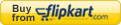Author Quantitative Aptitude For Competitive Examinations (S.Chand) R.S.Agarwal Quantum CAT (Arihant) Sarvesh Kumar Fast Track Objective Arithmetic (Arihant) Rajesh Verma
• The point is, both books of Arihant Publication (Sarvesh or Rajesh) are way better than R.S.Agarwal, in terms of content, presentation, language and coverage, without being too expensive than R.S.Agarwal’s book.
• And both of them have universal application for almost all of the competitive exams in India (for maths segment).
• My advice, go with either Rajesh Verma or Sarvesh Kumar. Then the question, which one to pick up?

## Fast Track Objective Arithmetic by Rajesh Verma.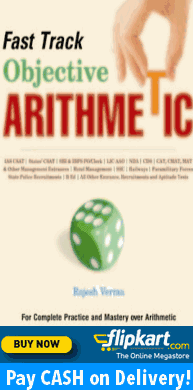## Quantam CAT by Sarvesh Kumar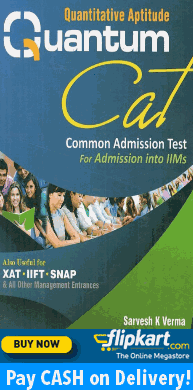• The size and price factor goes in favor of this book.
• Concepts, techqniues, readymade formulas given here and there. Language, presentation is lucid.
• Algebra, quadratic equation and Trigonometry specific chapters are given for exclusively for SSC.
• If you solve all the sums of this book, then mathematics portion of SSC-CGL (Tier-I and II) will be as easy as a walk in the park.
• Although book is written for CAT and  Management exams, he starts explaining everything from basics. Then exercises are divided into “Introductory<level 1<Level 2<Final round”, based on difficulty level.
• Thus it becomes ideal choice for any aptitude exam.
• So for lower level exams (SSC/IBPS), you should solve all his solved examples, then introductory exercises, finally level 1.
• That’ll be quite sufficient.
• While it is excellent for SSC, IBPS, UGC, LIC, CDS etc. level exams, its utility starts diminishing as you move towards higher-end exams.
• Function, graphs, Quadratic equations, Geometry, Permutation-Combination-Probability coverage is “Thorough”. (Hell even more sums than Arun Sharma’s).
• This make it ideal “text-book” for CAT/CMAT and other higher end exams.

^But merely getting either of these books, is not going to make you a topper.

If you want to become truly “invincible” for the maths portion of any competitive exam, then you must practice maximum numbers of questions at home and maintain a “diary of mistakes”.

This concludes how to approach Mathematics/Quantiative Aptitude for SSC-CGL exam.

Now only two topics remain (will be discussed later, in separate articles):

1. How to approach Logical Reasoning
2. How to approach English vocabulary, grammar and comprehension